# Wave equation diagram problem

## Homework Statement

The question is to sketch for values of t = 0, t =0.25, t = 0.5, t=1 and t = 1.25 for the wave equation

Ytt = Yxx in the infinite domain with initial conditions

y(x,0) = f(x) =
\begin{cases}
1 & \text{if } 0 ≤ x ≤ 1 \\
& 0 (otherwise)
\end{cases}

Yt(x,0) = 0

## Homework Equations

Y(x,t) = a(x,t) + b(x,t)

where a(x,t) = ## \frac {1} {2} \ ## f(x+t)
and b(x,t) = ## \frac {1} {2} \ ## f(x-t)

## The Attempt at a Solution

These are the sketches I obtained for those times and they are not to exactly measured to the points as I did them in paint. Can someone please tell me if the sketches are correct for this question!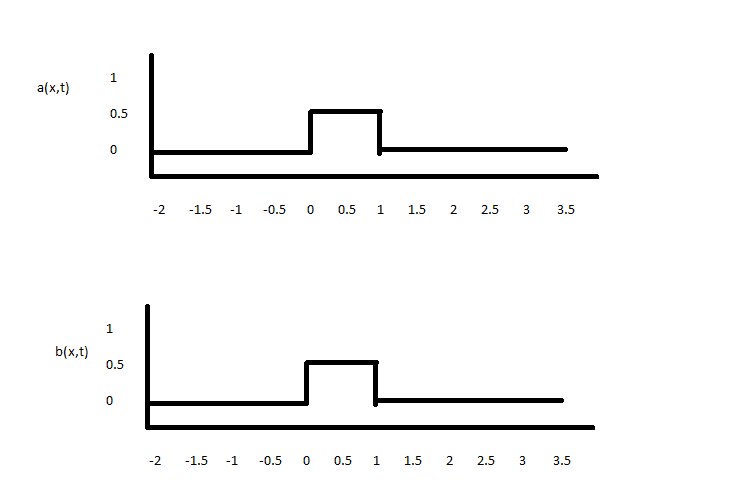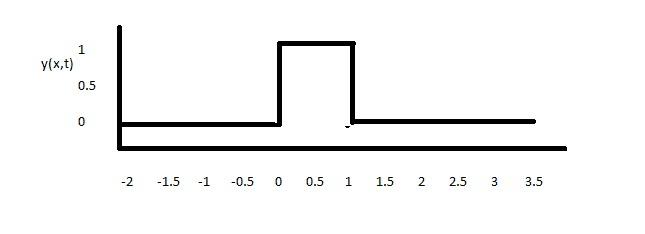for t =0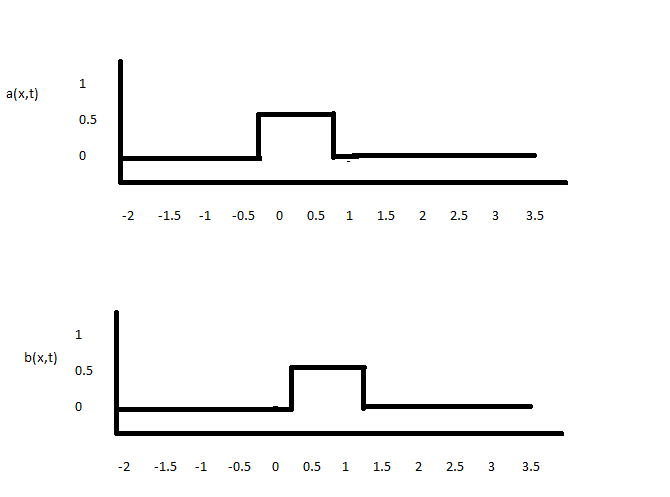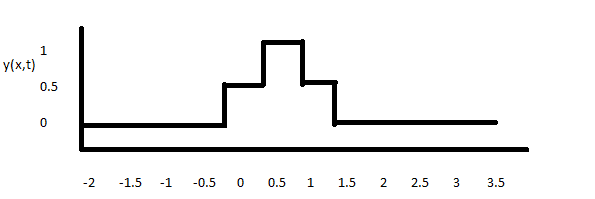for t= 0.25

Attaching the remaining ones in the post below as you can't attach more than 6 in one post.

#### Attachments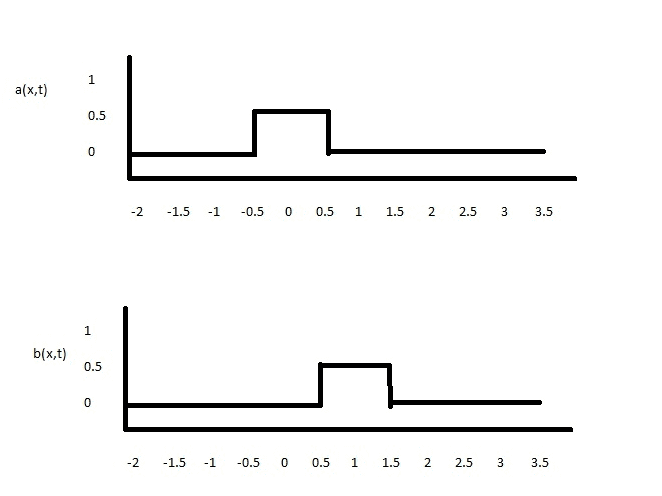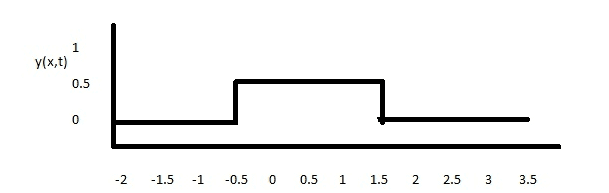for t = 0.5 s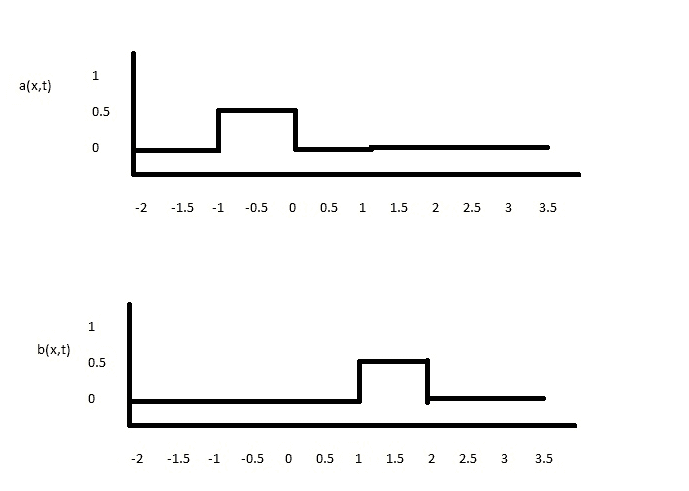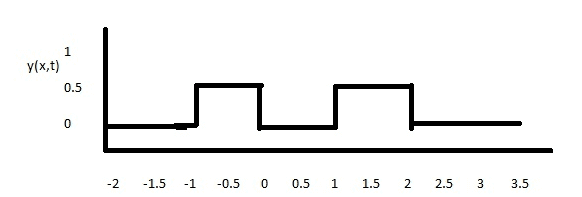for t = 1 s

BvU
Homework Helper
Hi JI,

As you may know, PF has great difficulty handing out approval stamps: it could create big problems with teachers, exam committees and what have you. PF homework is meant to help folks when they get stuck; with hints, questions and advice. My personal opinion is you don't need any of those :)

and ;)

Hi JI,

As you may know, PF has great difficulty handing out approval stamps: it could create big problems with teachers, exam committees and what have you. PF homework is meant to help folks when they get stuck; with hints, questions and advice. My personal opinion is you don't need any of those :)

and ;)

Look man can you be more clear? I don't understand what are you talking about. All I asked is if my sketches are correct or not, I am not asking you to do my homework for me.

BvU
Homework Helper
Yes I can be more clear: I think what you did is excellent -- but you can't derive any rights from my statements.

My post #3 tries to express some reluctance about the "Is this correct" questions.

Yes I can be more clear: I think what you did is excellent -- but you can't derive any rights from my statements.

My post #3 tries to express some reluctance about the "Is this correct" questions.

Cheers mate! I just realized I missed out t= 1.25 s for this question

Can you see the sketches for t = 1.25 s are excellent as well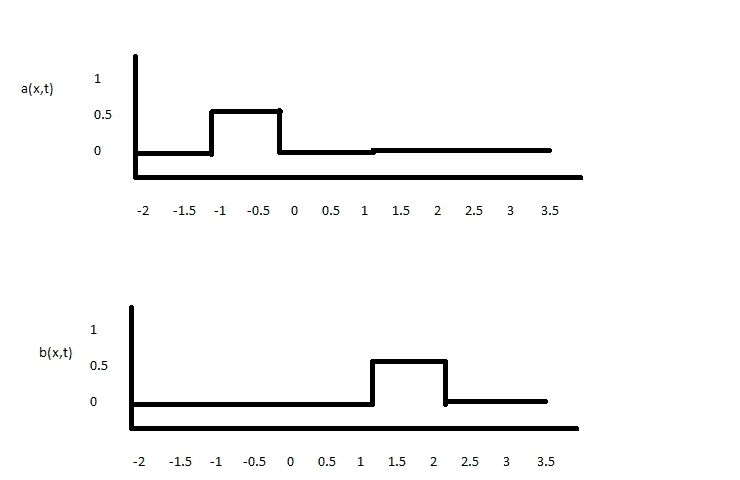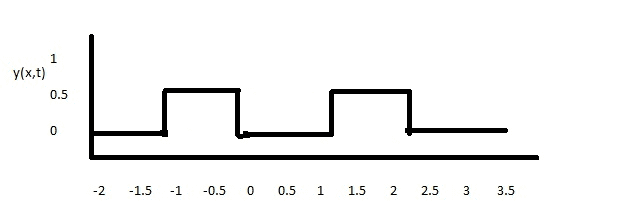BvU